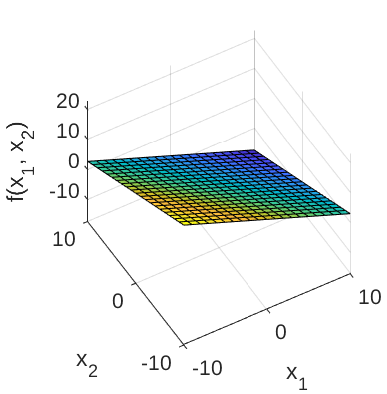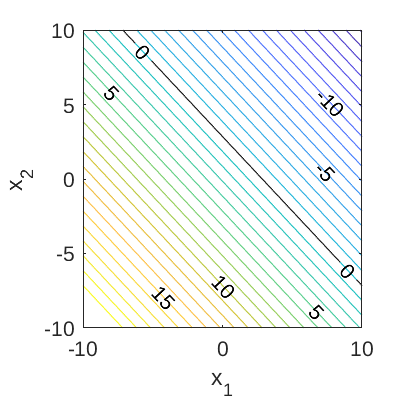High-dimensional Example Function

The high-dimensional limit state function is used to test algorithms in structural reliability analysis, such as Adaptive Kriging Monte Carlo simulation (AK-MCS) and others (Rackwitz, 2001). A prominent feature of this test function is that the failure probability does not change significantly when changing the number of input dimensions.

Description

The analytic expression of the high-dimensional limit state function is given as:

g(\mathbf{x}) = \left(M + 3 a \sqrt{M}\right) - \sum_{i=1}^{M} x_i,

where M is the number of input variables (or dimensions; positive integer); \{x_m\}_{m=1}^{M} are the input variables; and a is a parameter. By default, the parameter is set to 0.2.

The failure event is defined as g(\mathbf{x}) \leq 0 and the failure probability P_f = \mathbb{P}[g(\mathbf{x}) \leq 0]. Figure 1 and 2 show the surface and contour plots of the 2-dimensional high-dimensional function using the default values of the constants, respectively.
In Figure 2, the limit state function at which g(\mathbf{x}) = 0 is highlighted.Figure 1: Surface plot of the 2-dimensional high-dimensional limit state function.Figure 2: Contour plot of the 2-dimensional high-dimensional limit state function. The limit state function at which g(\mathbf{x})=0 is also shown.

Inputs

M independent identically distributed lognormal random variables are considered for the high-dimensional limit state function.

No Variable Distribution Parameters
1 x_1 Lognormal \mu_{x_1} = 1,
\sigma_{x_1} = 0.2
\vdots \vdots \vdots \vdots
M x_M Lognormal \mu_{x_M} = 1,
\sigma_{x_M} = 0.2

Parameter

By default, the parameter a is set to 0.2 following Rackwitz (2001).

Reference values

The asymptotic value of the failure probability P_f with M \rightarrow \infty is 1.35 \times 10^{-3} (Rackwitz, 2001). Some other values of P_f calculated using different methods for different values of M are shown in the table below.

Method M N \hat{P}_f \text{CoV}[\hat{P}_f] Source
FORM 50 154 1.531 \times 10^{-4} - Rackwitz (2001)
SORM 50 1'480 2.555 \times 10^{-3} - Rackwitz (2001)
MCS 50 10^{6} 1.915 \times 10^{-3} 2.28\% UQLab v1.2.1
FORM 100 304 3.369 \times 10^{-4} - Rackwitz (2001)
SORM 100 5'455 2.98 \times 10^{-3} - Rackwitz (2001)
MCS 100 10^{6} 1.685 \times 10^{-3} 2.43\% UQLab v1.2.1
FORM 100 603 6.212 \times 10^{-6} - Rackwitz (2001)
SORM 200 20'904 4.53 \times 10^{-3} - Rackwitz (2001)
MCS 200 10^{6} 1.669 \times 10^{-3} 2.45\% UQLab v1.2.1

Resources

The vectorized implementation of the high-dimensional limit state function in MATLAB as well as the script file with the model and probabilistic inputs definitions for the function in UQLAB can be downloaded below:

uq_highDimensional.zip (2.4 KB)

The contents of the file are:

Filename Description
uq_highDimensional.m vectorized implementation of the high-dimensional limit state function in MATLAB
uq_Example_highDimensional.m definitions for the model and probabilistic inputs in UQLab
LICENSE license for the function (BSD 3-Clause)

Reference

• R. Rackwitz, “Reliability analysis—a review and some perspectives,” Structural Safety, vol. 23, no. 4, pp. 365–395, 2001. DOI:10.1016/S0167-4730(02)00009-7